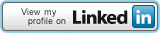## Connect Me## Pages

### QBASIC Programs for Grade 5 to 8

WAP to enter US\$, convert it into Nepali currency and print it.
CLS
INPUT "Enter US \$ value"; a
b=n * 85
PRINT "Nepali currency of supplied US\$ is "; b
END

WAP to enter a number and print the square and cube of it.
CLS
INPUT "Enter a number"; p
Sq= p * p
Cu= p * p * p
PRINT "Square value is "; Sq
PRINT "Cube value is "; Cu
END

WAP to find loss amount, if CP is Rs. 10000 and SP is Rs. 8000.
CLS
LET cp=10000
LET sp=8000
l=cp-sp
PRINT "Loss amount is "; l
END

WAP to find Simple Interest, after taking Principal, Interest and Rate from user.
CLS
INPUT "Enter Principal"; p
INPUT "Enter Time"; t
INPUT "Enter Rate"; r
si=(p*t*r)/100
PRINT "Simple interest"; si
END

WAP to find the final amount the user has to pay, if the shopkeeper allows 10% discount on total sales for 5 chairs at the rate of Rs 650 each.
CLS
LET ch=5
LET rate=650
LET total=ch*rate
LET dis=total * (10/100)
fa=total-dis
PRINT "Final amount to pay "; fa
END

WAP to find the breadth of a rectangle, if area is 25 m2 and length is 5m.
CLS
LET l=5
LET area=25
LET b=area/l
END

WAP to ask roll number, full name, grade and section of a student and print them sequentially.
CLS
INPUT "Enter your roll number "; rn
INPUT "Enter your full name"; fn\$
PRINT "Your roll number is"; rn
PRINT "Your full name is ";fn\$
END

WAP to ask time in minute and convert it into second.
CLS
INPUT "Enter time in minute"; m
sec=m*60
PRINT "Time in second is " ; sec
END

WAP to ask a number and print whether the number is EVEN or ODD number.
CLS
INPUT "Enter a number "; x
r= x MOD 2
IF r=0 then
PRINT "The supplied number is even"
ELSE
PRINT "The supplied number is odd"
END IF
END

WAP to find the distance travelled by a car, if it travels continuously for 8 hours with the speed of 95 km/hr.
CLS
LET hr=8
LET sp=95
LET dis=hr*sp
PRINT "Total distance travelled by a car"; dis
END

WAP to convert 5500 grams into kilograms.
CLS
LET gm=5500
LET kg=gm/1000
PRINT "The value in kilogram is "; kg
END

WAP to display the name of places you visited during Dashain and Tihar.
CLS
LET d\$="Ilam"
LET d1\$="Jhapa"
LET d2\$="Pokhara"
LET d3="Manakamana"
LET d4\$="Sauraha"
LET d5\$="Jomsom"
PRINT "The places I visited are"; d\$, d1\$, d2\$, d3\$, d4\$, d5\$
END

WAP to enter and display your favourites.
CLS
INPUT "Enter your fav. Actor name"; a\$
INPUT "Enter your fav. Movie"; m\$
INPUT "Enter your fav. Footballer"; f\$
INPUT "Enter your fav. Jersey number"; j
INPUT "Enter your fav. Football club"; fc\$
INPUT "Enter your fav. Singer"; s\$
INPUT "Enter your fav. Book"; b\$
PRINT a\$, m\$, f\$, j, fc\$, s\$, b\$
END

WAP to enter marks of 5 different subjects and print total mark and average mark.
CLS
INPUT "Enter marks of English"; a
INPUT "Enter marks of Nepali "; b
INPUT "Enter marks of Maths"; c
INPUT "Enter marks of Science"; d
INPUT "Enter marks of Computer"; e
tot=a+b+c+d+e
av=tot/5
PRINT "Total marks "; tot
PRINT "Average mark"; av
END

Ą